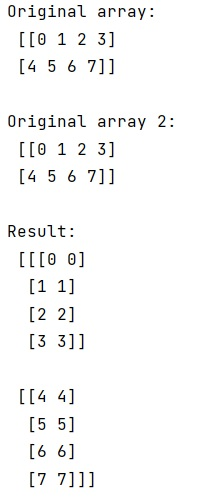# How to zip two 2D NumPy arrays?

Given two 2D NumPy arrays, we have to add zip them.
Submitted by Pranit Sharma, on March 05, 2023

## Zip two 2D NumPy arrays

Suppose that we are given two 2D numpy arrays and we need to zip these arrays so that we can get the values of both arrays together along with the corresponding map value.

For example, if we have [[1,2,3],[4,5,6]] and [[1,2,3],[4,5,6]] as two numpy arrays and we need to zip them together.

To zip two 2D NumPy arrays, we can use the numpy.dstack() method. This method stack arrays in sequence depth-wise. This can be considered as concatenation along the third axis after 2-D arrays of shape (M, N) have been reshaped to (M, N,1).

Let us understand with the help of an example,

## Python code to zip two 2D NumPy arrays

```# Import numpy
import numpy as np

# Creating two numpy arrays
arr = np.array([[0, 1, 2, 3],[4, 5, 6, 7]])
arr1 = np.array([[0, 1, 2, 3],[4, 5, 6, 7]])

# Display Original arrays
print("Original array:\n",arr,"\n")
print("Original array 2:\n",arr1,"\n")

# Applying dstack on arrays
res = np.dstack((arr,arr1))

# Display result
print("Result:\n",res,"\n")
```

Output:Languages: » C » C++ » C++ STL » Java » Data Structure » C#.Net » Android » Kotlin » SQL
Web Technologies: » PHP » Python » JavaScript » CSS » Ajax » Node.js » Web programming/HTML
Solved programs: » C » C++ » DS » Java » C#
Aptitude que. & ans.: » C » C++ » Java » DBMS
Interview que. & ans.: » C » Embedded C » Java » SEO » HR
CS Subjects: » CS Basics » O.S. » Networks » DBMS » Embedded Systems » Cloud Computing
» Machine learning » CS Organizations » Linux » DOS
More: » Articles » Puzzles » News/Updates